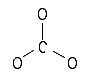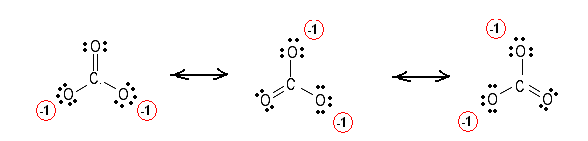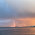Simple method for writing Lewis Structures – Ozone O3 and carbonate CO3-2 | Chemistry Net

# Simple method for writing Lewis Structures – Ozone O3 and carbonate CO3-2

Simple method for writing Lewis structures - Ozone O3 and carbonate CO3-2

# Simple Method for writing Lewis Electron Dot Structures - Ozone O3 and carbonate CO3-2

A simple method for writing Lewis electron dot structures is given in a previous article entitled “Lewis Structures and the Octet Rule”. Examples for writing Lewis structures following the above procedure are given bellow:

Step1: The central atom will be one of the oxygen atoms.  Connect the 3 atoms with a single bonds

O – O – O

Step 2: Calculate the # of electrons in p bonds (pi bonds, multiple bonds) using formula (1) in the article entitled “Lewis Structures and the Octet Rule”.

Where n in this case is 3 since O3 consists of three atoms

Where V = (6 + 6 + 6 )  = 18

Therefore, P = 6n + 2 – V = 6 * 3 + 2 – 18 = 2      Therefore, there are 2 π electrons (pi electrons) in O3  and so 1 double   bond must be added to the structure of Step 1.

Step 3 & 4:  The 2 atoms are joined together with a double bond. Therefore the Lewis electron dot structures for O3 are as follows:

﻿﻿Carbonate species act as buffers and are necessary for all biological systems. They also play important role in neutralization of strong acids and bases. The carbonate system as it is called is the major source of buffering in the ocean and in natural waters.

Step1: The central atom will be the C atom since it is the only atom with “subscript” equal to 1 in the molecular formula.  Connect the O atoms with the C atom with single bonds.Step 2: Calculate the # of electrons in π bonds (pi bonds, multiple bonds) using  formula(1) in the article entitled “Lewis Structures and the Octet Rule”.:

Where n in this case is 4 since CO3-2consists of four atoms

Where V = (4 + 6 + 6 + 6 ) – (-2)  = 24   Therefore, P = 6n + 2 – V = 6 * 4 + 2 – 24 = 2

There are 2 π electrons (pi electrons) in  CO3-2  and therefore 1 double  bond must be added to the structure of Step 1.

Step 3 & 4: One double bond between C and O is added to the structure in step 1. Unshared electron pairs are added so that there is an octet of electrons around each atom. All the equivalent resonance structures are drawn by delocalizing electron pairs. Therefore, the Lewis electron dot structures for CO3-2 are as follows:Figure 4: Lewis structures for the carbonate ion CO3-2. The 3 structures are equivalent since they have equal formal charges on the elements. It has been proven experimentally that the C-O bond in the carbonate ion is a hybrid of a single and a double bond (the length of the C-O bond in the carbonate ion is approximately half of the sum of the lengths of a normal C-O and C=O bond).This means that the theoretical Lewis structures are in agreement with experimental results.

Relevant Posts

Lewis Structures and the Octet Rule - A simple method to write Lewis Structures

Simple Procedure for writing Lewis Structures- CO2, NCO

Lewis dot structures - Electron dot structure of CO

Lewis Dot Structure of Carbonic Acid H2CO3

Simple Method for drawing Lewis structures (Video): H2CO3

References

1. G.N. Lewis, J.A.C.S, 38, 762-785, (1916)
2.  E. C. McGoran, J. Chem. Educ., 68, 19-23 (1991)
3. A.B.P. Lever, J. Chem. Educ., 49, 819-821, (1972)

Key Terms

electron dot Lewis structures, Lewis Structures and the Octet Rule, how can we draw the carbonate Lewis dot structure

#### 5 comments:

1.how to do it for CH4

2.why we should put double bond instead of full lone pair at O?

1.Structures with large charge separation are unstable. This means that are minor contributors to the resonance. If you put a lone pair the charge separation is larger and you draw a minor contributor.

3.This is most helpful for me
Thanks

4.How to place unshared electrons after calculation of P(formula for multiple bond determination )?